Thermodynamics and Propulsion

# 3.3 The Carnot Cycle

A Carnot cycle is shown in Figure 3.4. It has four processes. There are two adiabatic reversible legs and two isothermal reversible legs. We can construct a Carnot cycle with many different systems, but the concepts can be shown using a familiar working fluid, the ideal gas. The system can be regarded as a chamber enclosed by a piston and filled with this ideal gas.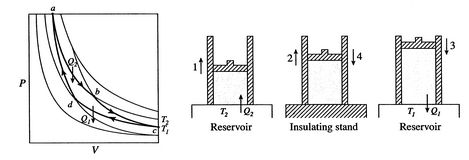The four processes in the Carnot cycle are:

1. The system is at temperature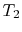at state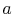. It is brought in contact with a heat reservoir, which is just a liquid or solid mass of large enough extent such that its temperature does not change appreciably when some amount of heat is transferred to the system. In other words, the heat reservoir is a constant temperature source (or receiver) of heat. The system then undergoes an isothermal expansion fromto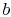, with heat absorbed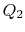.
2. At state, the system is thermally insulated (removed from contact with the heat reservoir) and then let expand to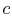. During this expansion the temperature decreases to. The heat exchanged during this part of the cycle,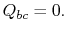)
3. At statethe system is brought in contact with a heat reservoir at temperature. It is then compressed to state, rejecting heat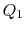in the process.
4. Finally, the system is compressed adiabatically back to the initial state. The heat exchange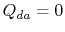.

The thermal efficiency of the cycle is given by the definition(3..4)

In this equation, there is a sign convention implied. The quantities,as defined are the magnitudes of the heat absorbed and rejected. The quantities,on the other hand are defined with reference to heat received by the system. In this example, the former is negative and the latter is positive. The heat absorbed and rejected by the system takes place during isothermal processes and we already know what their values are from Eq. (3.1):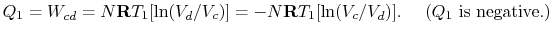The efficiency can now be written in terms of the volumes at the different states as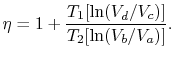(3..5)

The path from statestoand fromtoare both adiabatic and reversible. For a reversible adiabatic process we know that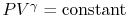. Using the ideal gas equation of state, we have. Along curve-, therefore,. Along the curve-,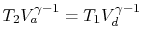. Thus,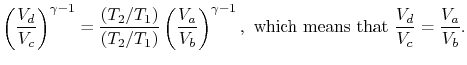Comparing the expression for thermal efficiency Eq. (3.4) with Eq. (3.5) shows two consequences. First, the heats received and rejected are related to the temperatures of the isothermal parts of the cycle by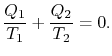(3..6)

Second, the efficiency of a Carnot cycle is given compactly by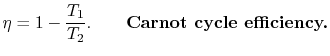(3..7)

The efficiency can be 100% only if the temperature at which the heat is rejected is zero. The heat and work transfers to and from the system are shown schematically in Figure 3.5.Muddy Points

Since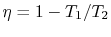, looking at the-graph, does that mean the farther apart the,isotherms are, the greater efficiency? And that if they were very close, it would be very inefficient? (MP 3.2)

In the Carnot cycle, why are we only dealing with volume changes and not pressure changes on the adiabats and isotherms? (MP 3.3)

Is there a physical application for the Carnot cycle? Can we design a Carnot engine for a propulsion device? (MP 3.4)

How do we know which cycles to use as models for real processes? (MP 3.5)

UnifiedTP